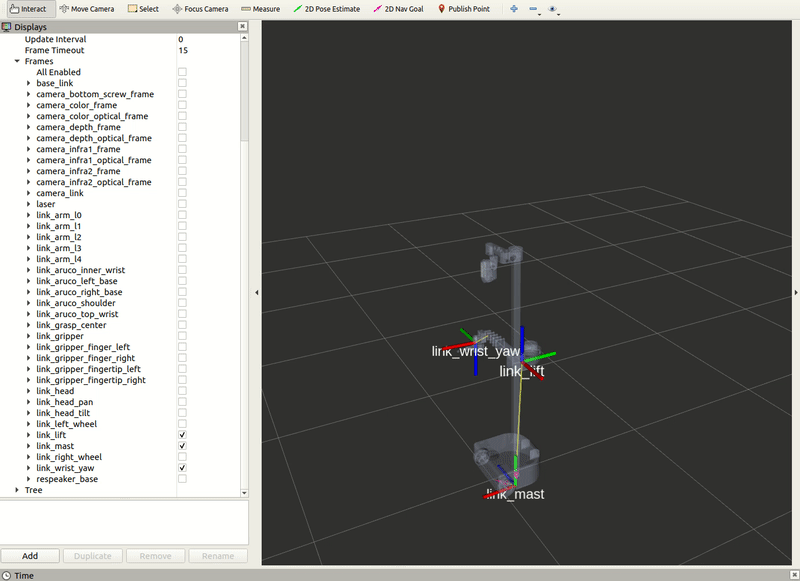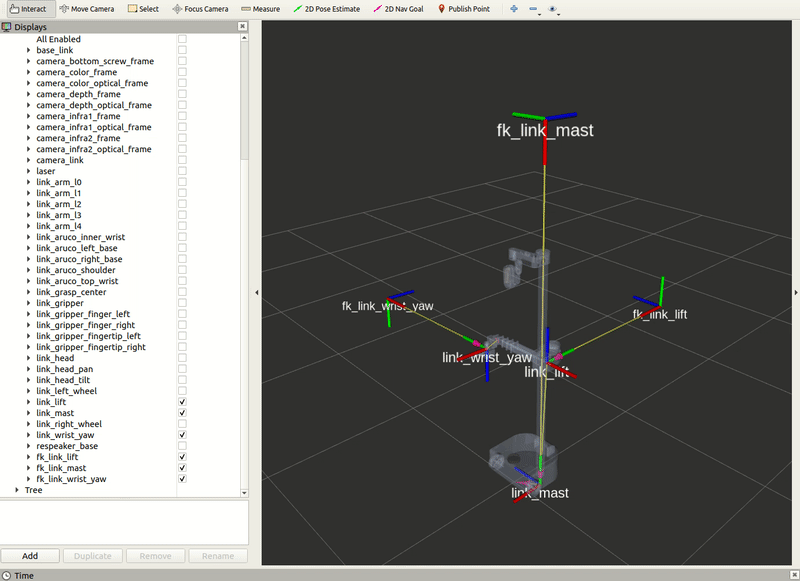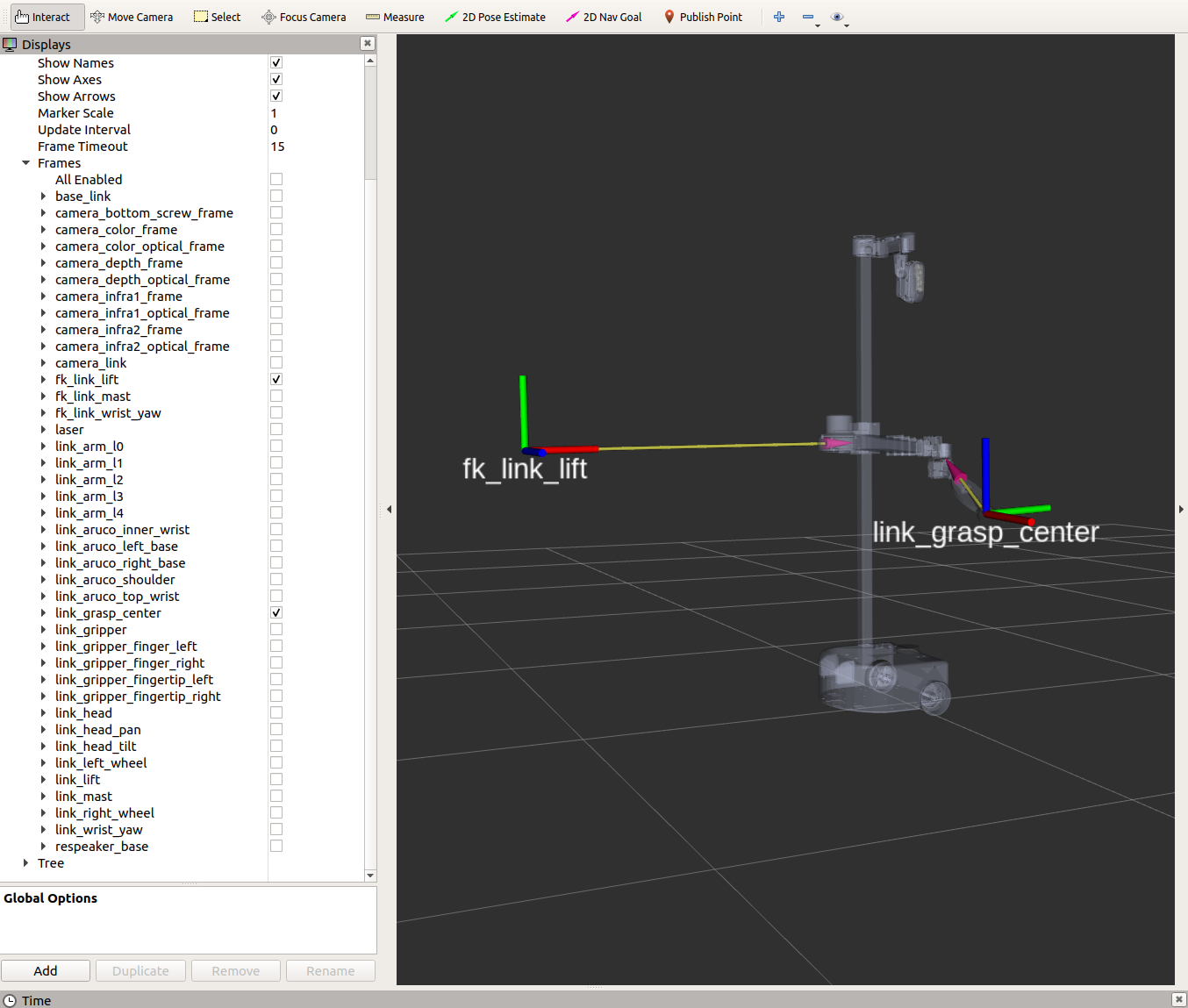# Example 10

This tutorial we will explain how to create a tf2 static broadcaster and listener.

For the tf2 static broadcaster node, we will be publishing three child static frames in reference to the `link_mast`, `link_lift`, and `link_wrist_yaw` frames.

Begin by starting up the stretch driver launch file.

``````roslaunch stretch_core stretch_driver.launch
``````

Within this tutorial package, there is an RViz config file with the topics for the transform frames in the Display tree. You can visualize these topics and the robot model by running the command below in a new terminal.

``````rosrun rviz rviz -d /home/hello-robot/catkin_ws/src/stretch_tutorials/rviz/tf2_broadcaster_example.rviz
``````

Then run the tf2_broadcaster.py node to visualize three static frames. In a new terminal, execute:

``````cd catkin_ws/src/stretch_tutorials/src/
``````

The GIF below visualizes what happens when running the previous node.Tip

If you would like to see how the static frames update while the robot is in motion, run the stow_command_node.py and observe the tf frames in RViz.

In a terminal, execute:

``````cd catkin_ws/src/stretch_tutorials/src/
python3 stow_command.py
``````### The Code

``````#!/usr/bin/env python3

import rospy
import tf.transformations
from geometry_msgs.msg import TransformStamped

"""
This node publishes three child static frames in reference to their parent frames as below:
"""
def __init__(self):
"""
A function that creates a broadcast node and publishes three new transform
frames.
:param self: The self reference.
"""

self.mast = TransformStamped()
self.mast.transform.translation.x = 0.0
self.mast.transform.translation.y = 2.0
self.mast.transform.translation.z = 0.0
q = tf.transformations.quaternion_from_euler(1.5707, 0, -1.5707)
self.mast.transform.rotation.x = q
self.mast.transform.rotation.y = q
self.mast.transform.rotation.z = q
self.mast.transform.rotation.w = q

self.lift = TransformStamped()
self.lift.transform.translation.x = 0.0
self.lift.transform.translation.y = 1.0
self.lift.transform.translation.z = 0.0
q = tf.transformations.quaternion_from_euler(1.5707, 0, -1.5707)
self.lift.transform.rotation.x = q
self.lift.transform.rotation.y = q
self.lift.transform.rotation.z = q
self.lift.transform.rotation.w = q

self.wrist = TransformStamped()
self.wrist.transform.translation.x = 0.0
self.wrist.transform.translation.y = 1.0
self.wrist.transform.translation.z = 0.0
q = tf.transformations.quaternion_from_euler(1.5707, 0, -1.5707)
self.wrist.transform.rotation.x = q
self.wrist.transform.rotation.y = q
self.wrist.transform.rotation.z = q
self.wrist.transform.rotation.w = q

self.br.sendTransform([self.mast, self.lift, self.wrist])

rospy.loginfo('Publishing TF frames. Use RViz to visualize')

if __name__ == '__main__':
rospy.spin()
``````

### The Code Explained

Now let's break the code down.

``````#!/usr/bin/env python3
``````
Every Python ROS Node will have this declaration at the top. The first line makes sure your script is executed as a Python3 script.

``````import rospy
import tf.transformations
from geometry_msgs.msg import TransformStamped
``````

You need to import rospy if you are writing a ROS Node. Import `tf.transformations` to get quaternion values from Euler angles. Import the `TransformStamped` from the `geometry_msgs.msg` package because we will be publishing static frames and it requires this message type. The `tf2_ros` package provides an implementation of a `tf2_ros.StaticTransformBroadcaster` to help make the task of publishing transforms easier.

``````def __init__(self):
"""
A function that creates a broadcast node and publishes three new transform
frames.
:param self: The self reference.
"""
``````

Here we create a `TransformStamped` object which will be the message we will send over once populated.

``````self.mast = TransformStamped()
``````

We need to give the transform being published a timestamp, we'll just stamp it with the current time, `rospy.Time.now()`. Then, we need to set the name of the parent frame of the link we're creating, in this case `link_mast`. Finally, we need to set the name of the child frame of the link we're creating. In this instance, the child frame is `fk_link_mast`.

``````self.mast.transform.translation.x = 0.0
self.mast.transform.translation.y = 2.0
self.mast.transform.translation.z = 0.0
``````

Set the translation values for the child frame.

``````q = tf.transformations.quaternion_from_euler(1.5707, 0, -1.5707)
self.wrist.transform.rotation.x = q
self.wrist.transform.rotation.y = q
self.wrist.transform.rotation.z = q
self.wrist.transform.rotation.w = q
``````

The `quaternion_from_euler()` function takes in an Euler angle as an argument and returns a quaternion. Then set the rotation values to the transformed quaternions.

This process will be completed for the `link_lift` and `link_wrist_yaw` as well.

``````self.br.sendTransform([self.mast, self.lift, self.wrist])
``````

Send the three transforms using the `sendTransform()` function.

``````rospy.init_node('tf2_broadcaster')
``````

The next line, `rospy.init_node(NAME, ...)`, is very important as it tells rospy the name of your node -- until rospy has this information, it cannot start communicating with the ROS Master.

Note

The name must be a base name, i.e. it cannot contain any slashes "/".

Instantiate the `FixedFrameBroadcaster()` class.

``````rospy.spin()
``````

Give control to ROS. This will allow the callback to be called whenever new messages come in. If we don't put this line in, then the node will not work, and ROS will not process any messages.

## tf2 Static Listener

In the previous section of the tutorial, we created a tf2 broadcaster to publish three static transform frames. In this section, we will create a tf2 listener that will find the transform between `fk_link_lift` and `link_grasp_center`.

Begin by starting up the stretch driver launch file.

``````roslaunch stretch_core stretch_driver.launch
``````

Then run the tf2_broadcaster.py node in a new terminal to create the three static frames.

``````cd catkin_ws/src/stretch_tutorials/src/
``````

Finally, run the tf2_listener.py node in a separate terminal to print the transform between two links.

``````cd catkin_ws/src/stretch_tutorials/src/
python3 tf2_listener.py
``````

Within the terminal, the transform will be printed every 1 second. Below is an example of what will be printed in the terminal. There is also an image for reference of the two frames.

``````[INFO] [1659551318.098168]: The pose of target frame link_grasp_center with reference from fk_link_lift is:
translation:
x: 1.08415191335
y: -0.176147838153
z: 0.576720021135
rotation:
x: -0.479004489528
y: -0.508053545368
z: -0.502884087254
w: 0.509454501243
``````### The Code

``````#!/usr/bin/env python3

import rospy
from geometry_msgs.msg import TransformStamped
import tf2_ros

class FrameListener():
"""
This Class prints the transformation between the fk_link_mast frame and the
"""
def __init__(self):
"""
A function that initializes the variables and looks up a transformation
between a target and source frame.
:param self: The self reference.
"""
tf_buffer = tf2_ros.Buffer()
listener = tf2_ros.TransformListener(tf_buffer)

rospy.sleep(1.0)
rate = rospy.Rate(1)

while not rospy.is_shutdown():
try:
trans = tf_buffer.lookup_transform(to_frame_rel,
from_frame_rel,
rospy.Time())
rospy.loginfo('The pose of target frame %s with reference from %s is: \n %s', from_frame_rel, to_frame_rel, trans.transform)
except (tf2_ros.LookupException, tf2_ros.ConnectivityException, tf2_ros.ExtrapolationException):
rospy.logwarn(' Could not transform %s from %s ', to_frame_rel, from_frame_rel)

rate.sleep()

if __name__ == '__main__':
rospy.init_node('tf2_listener')
FrameListener()
rospy.spin()
``````

### The Code Explained

Now let's break the code down.

``````#!/usr/bin/env python3
``````
Every Python ROS Node will have this declaration at the top. The first line makes sure your script is executed as a Python3 script.

``````import rospy
from geometry_msgs.msg import TransformStamped
import tf2_ros
``````

You need to import `rospy` if you are writing a ROS Node. Import the `TransformStamped` from the `geometry_msgs.msg` package because we will be publishing static frames and it requires this message type. The `tf2_ros` package provides an implementation of a `tf2_ros.TransformListener` to help make the task of receiving transforms easier.

``````tf_buffer = tf2_ros.Buffer()
listener = tf2_ros.TransformListener(tf_buffer)
``````

Here, we create a `TransformListener` object. Once the listener is created, it starts receiving tf2 transformations and buffers them for up to 10 seconds.

``````from_frame_rel = 'link_grasp_center'
``````
Store frame names in variables that will be used to compute transformations.

``````rospy.sleep(1.0)
rate = rospy.Rate(1)
``````

The first line gives the listener some time to accumulate transforms. The second line is the rate at which the node is going to publish information (1 Hz).

``````try:
trans = tf_buffer.lookup_transform(to_frame_rel,
from_frame_rel,
rospy.Time())
rospy.loginfo('The pose of target frame %s with reference from %s is: \n %s', from_frame_rel, to_frame_rel, trans.transform)

except (tf2_ros.LookupException, tf2_ros.ConnectivityException, tf2_ros.ExtrapolationException):
rospy.logwarn(' Could not transform %s from %s ', to_frame_rel, from_frame_rel)
``````

Try to look up the transformation we want. Use a try-except block, since it may fail on any single call, due to internal timing issues in the transform publishers. Look up transform between `from_frame_rel` and `to_frame_rel` frames with the `lookup_transform()` function.

``````rospy.init_node('tf2_listener')
FrameListener()
``````

The next line, `rospy.init_node(NAME, ...)`, is very important as it tells rospy the name of your node -- until rospy has this information, it cannot start communicating with the ROS Master.

Note

The name must be a base name, i.e. it cannot contain any slashes "/".

Instantiate the `FrameListener()` class.

``````rospy.spin()
``````

Give control to ROS. This will allow the callback to be called whenever new messages come in. If we don't put this line in, then the node will not work, and ROS will not process any messages.